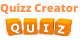Create Quiz

# Quiz: Algorithms Mock tests on Divide and Conquer.## The divide and conquer is an algorithm design paradigm based on -

single branched recursion
multi-branched recursion
uni-branched recursion

## The Divide and Conquer algorithm is similar to the Approach of

random programming
dynamic programming
slow programming

removed
modified
categorized

new sort
long sort
quick sort

## Which mathematical technique prove the correctness of a divide-and-conquer algorithm?

Value theory
mathematical induction
equation system

Sort/Unsort
Pluc/Minus
Divide/Break

## Which thing determines the computational cost of a divide-and-conquer algorithm?

infinite relations
submission & relations
recurrence relations

objects
optimal solution
list

6
3
2

## Which is an example of divide and conquer algorithm?

The Value Search
The Binary Search
The Limit search

## The name decrease and conquer has been proposed instead for

the single-subproblem class
the equation problem class
the ordinary class

## Which is an ancient decrease-and-conquer algorithm?

Bull algorithm
Euclidean algorithm
Nelian algorithm

## The divide-and-conquer paradigm often helps in the discovery of -

files
efficient algorithms

analog processor
single-processor
multi-processor

## Divide-and-conquer algorithms naturally tend to make efficient use of -

history
ALU
memory caches

Currently, we have no comments. Be first to comment on this quiz.

SIMILAR QUIZZESEcology Quiz: Interactions Of Living Things Quiz 2
Below is an Ecology Quiz on Interactions of Living Things. In an environment living and nonliving things interact at one point as they each have something that the other will need. Their interactio...Quiz: Data Structures Mock tests on Array
This quiz set contains information about Data Structures Array. Data Structure is a way to store and organize data so that it can be used efficiently. Our Data Structure tutorial includes all topic...Watergate Scandal History Quiz
The Watergate scandal was a major political scandal that occurred in the United States during the early 1970s. Let's learn more things together!

## Quiz: Algorithms Mock tests on Divide and Conquer. : Test Trivia

Ultimate impossible quiz game

Embed This Quiz
Copy the code below to embed this quiz